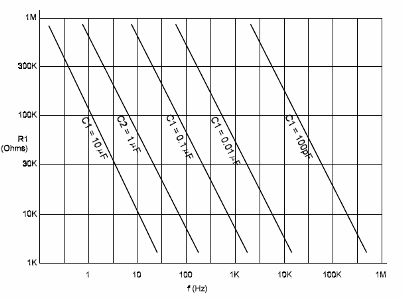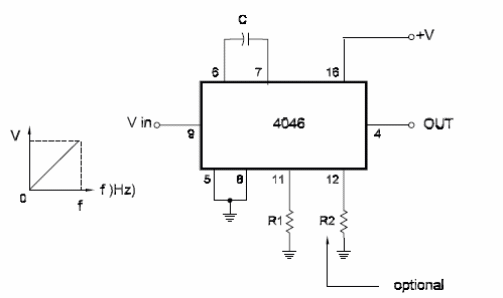## Voltage-Controlled Oscillator [VCO] Using the 4046 (CB109)

A VCO or VCF [Voltage to Frequency Converter] can be built using part of a 4048 [PLL] circuit. The basic circuit is shown here. This circuit can be used to produce signals in a frequency range of 100:1 to 1000’] cf based on the components used as reference. The configuration uses the VCO existing inside the 4048 that can operate in frequencies up to 1.6 MHz when powered from 15 V supplies.

The basic frequency for this oscillator is determined by C1 and R1. Without any other components, the operation frequency range will vary from the operating frequency when a control voltage of O V is applied to the input, to twice this frequency when the input voltage is +V [power-supply voltage]. This range can be shifted with the aid of a second resistor [R2].The new range of frequencies can be found by the curves shown bellow.R2 will raise the range in direct proportion to its value when compared to R1. For instance, if the range will change from 0 kHz to 1 kHz without R2, and R1 is 10 k ohm, then when inserting a 20 k ohm for R2, the range will be changed to 0,5 to 1.5 kHz. Other important characteristics for this circuit are the input impedance of 105 M ohm and operating current of only 90 µA when the circuit is powered from a 10 V source.Voltage-Controlled Oscillator [VCO] Using the 4046

Datasheet of 4046